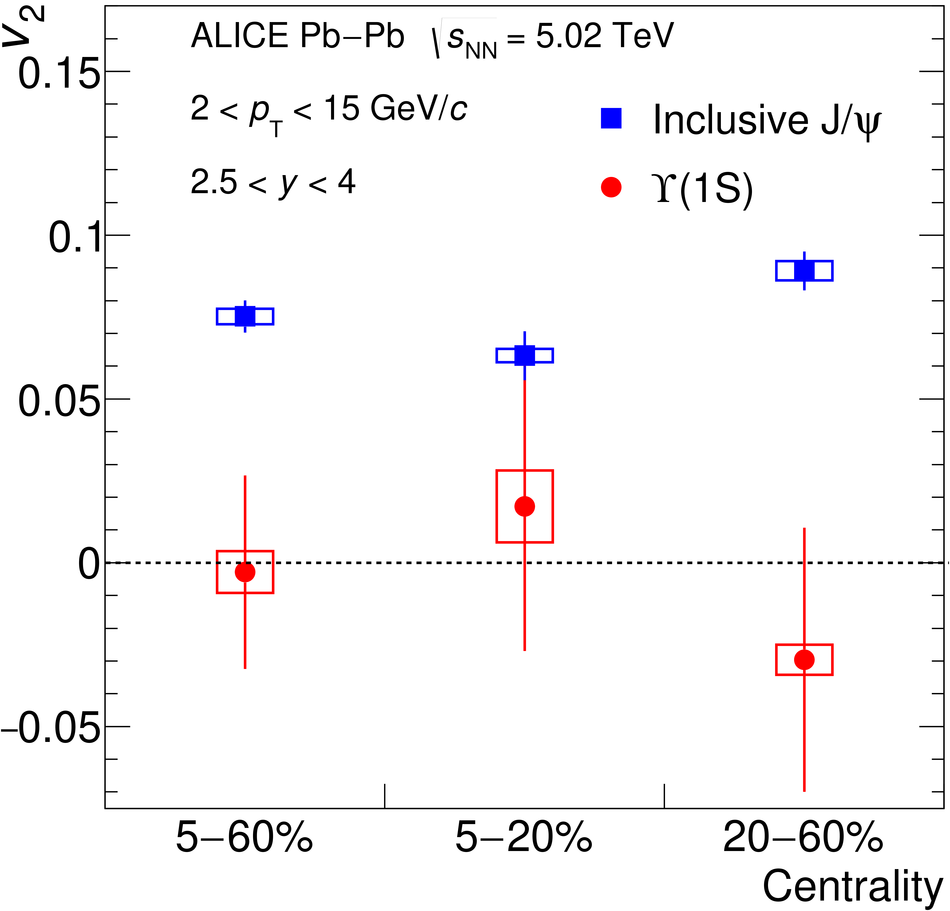# Figure 3

 The $\Upsilon$(1S) $v_2$ coefficient integrated over the transverse momentum range 2 $< \pt <$ 15 GeV/$c$ in three centrality intervals compared to that of inclusive ${\rm J}/\psi$. Error bars (open boxes) represent the statistical (systematic) uncertainties.